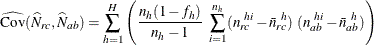# The SURVEYFREQ Procedure

#### Covariances of Frequency Estimates

The covariance matrix of the table cell frequency estimates is anmatrix that contains the pairwise cell frequency covariances.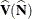denotes the covariance matrix and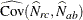denotes the pairwise covariances, for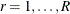;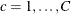;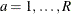; and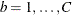.

PROC SURVEYFREQ estimates the covariances by using the variance estimation method that you request. If you request BRR variance estimation (by specifying the VARMETHOD=BRR option in the PROC SURVEYFREQ statement), PROC SURVEYFREQ estimates the covariances by using the BRR method. If you request jackknife variance estimation (by specifying the VARMETHOD=JACKKNIFE option), PROC SURVEYFREQ estimates the covariances by using the jackknife method. For more information, see the sections Balanced Repeated Replication (BRR) and The Jackknife Method.

By default, or if you request Taylor series variance estimation, PROC SURVEYFREQ estimates the covariance between frequency estimates for table cells (r,c) and (a,b) as